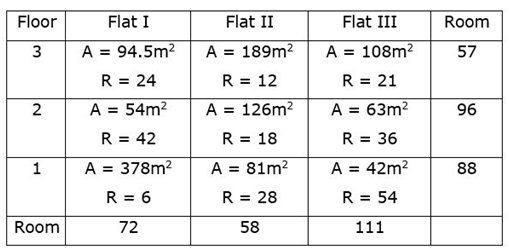Flat Based Puzzle Questions for PO Mains Floor with Questions

Flat Based Puzzle Questions for PO Mains Floor with Questions

Dear Aspirants, Our IBPS Guide team is providing new series of reasoning questions For SBI/IBPS PO Mains So that candidates can practice it on daily basis. These questions are designed by our skilled experts after thoroughly understanding your requirements. Aspirants can practice these new series of questions daily to get familiar with the exact exam pattern and make your preparation effective.

start quiz

Direction (1-3): Study the information carefully and answer the questions given below.

There are three floors in a given building such that floor 2 is above floor 1 and floor 3 is above floor 2. In the building, there are three flats on each floor such that flat A is to the west of flat B, which is to the west of flat C.

In the building, the area of ​​each flat on each floor is 2520 feet and each flat has certain number of rooms and no two flats have the same number of rooms.

The area of ​​each room of a flat on an odd-numbered floor is 210 ft.

The 12-room floor is on one of the floors below the floor with each room area of ​​360 feet.

floor 3. but the area of ​​each roomthird The area of ​​flat A is 420 feet more than the area of ​​each room of a 12-room flat. The total number of rooms in all the flats on the second floor is 15. Number of rooms in second floor flat C is more than the number of rooms in second floor flat A but less than the number of rooms in second floor flat B. destination. The flat having each room area of ​​420 feet is to the west of the flat having each room area of ​​252 feet. There is only one floor between the floors which has 2 rooms and the area of ​​each room is 630 feet but in the same flat. The area of ​​each room is 504 feet in an even numbered flat. The flat with each room area of ​​315 feet is just below the 5 room flat.

1) What is the area of ​​a room in flat B on floor 2?

a.340

b.360

C.396

Deither a) or c)

I.None of these

2) What is the total number of rooms on the third floor?

a.22

b.21

C.20

D.18

I.None of these

3) Which of the following statement is true?

aThe total number of rooms on floor 2 is 16. Is

bArea of ​​each room 1. Flat A’s on the floor is 494 feet

CTotal rooms in flat B of all three floors are 20.

DThere are 9 total rooms in flat A of all the three floors.

Ino one is right

Direction (4-7):
Study the following information carefully and answer the questions given below.

Five banks viz. – SBI, PNB, BOB, HDFC and ICICI are in a five-floor building from bottom to top respectively such that the lowest floor is marked as 1 and the topmost floor is marked as 5. Three employees of each bank who have been nominated. Manager, Assistant Manager, and Clerk take three lifts I, II and III to move up or down to their respective floors.

pay attention:- None of the three persons from the same bank take the same lift. Also, a person holding the same designation on adjacent floors is not taking the same lift.

B, who is an assistant manager, works on an even numbered floor, takes lift II. H who works three floors above B and is neither an assistant manager nor takes the same lift as B. SBI clerk takes lift I and works three floors below L, who neither works in same bank with C nor takes lift III. H and L have same designation but not Assistant Manager. The one who is manager from ICICI is not taking lift III. E works on one of the floors below H and is designated as Manager. I and O work in the same bank with E, who does not take lift II. L and D who is a clerk work in the same bank. I and C have the same designation, but none of them carry lift III. C and K, who is an assistant manager, are working on the same floor. N works on the same floor as F, who takes lift II. G works as a manager and works three floors below J, who does not take the same lift as A.

4) What is the designation of A and the bank?

aManager – PNB

bclerk – bob

C.Assistant Manager – ICICI

D.SBI – Clerk

I.None of these

5) Four of the five are related to each other in a certain way and thus form a group, find which of the following is odd?

a.C

b.b

C.M

D.D

I.n

6) Who among the following works as manager in BOB?

a.I

b.h

Ccoverage

D.Yes

I.None of these

7) Who among the following persons are working in SBI?

acke

bGKF

CCGO

Damb

I.None of these

Direction (8-10): Study the following information carefully and answer the questions given below.

A building has three floors numbered 1 to 3 from bottom to top. On each floor there are three flats, Flat I, Flat II and Flat III, from west to east. The area of ​​each flat is 2268m. Is2, Each flat has different rooms and no flat has the same number of rooms.

The number of rooms from flat I of floor 2 is equal to the area of ​​each room from flat III of floor 1. The area of ​​each room is 63 m from flat II of floor 32 The area of ​​each room is greater than that of flat II on floor no. The total number of rooms on floor number 3 is 57. The total number of rooms in Flat I is 14 more than the total number of rooms in Flat II. The area of ​​each room from flat III of floor number 3 is twice the number of rooms in flat III of floor no. The number of rooms in flat II of floor 2 is 3/4th of the number of rooms in flat I of floor no. The area of ​​each room of flat I from floor number 3 is 94.5 m2, The number of rooms in the flat in which I have taken all the floors together is 72. The number of rooms in flat I of floor no.1 is the square root of the number of rooms in flat-III of floor no.2.

8) What is the difference between the area of ​​flat II on floor 3 and flat III on floor I?

a.117

b.108

C.72

D.126

I.None of these

9) How many rooms are there in flat III of floor 3?

a.36

b.21

C.54

D.18

I.None of these

10) What is the total number of rooms in flat III of all the floors?

a.108

b.96

C.111

D.126

I.58

Attempt Puzzle Questions for Online Mock Tests

Directions (1-3):

• It is given that the area of ​​each room of a flat on an odd-numbered floor is 210 ft.
• The 12-room floor is one of the floors below the floor, with each room having an area of ​​360 feet.
• floor 3. but the area of ​​each roomthird The area of ​​flat A is 420 feet more than the area of ​​each room of a 12-room flat.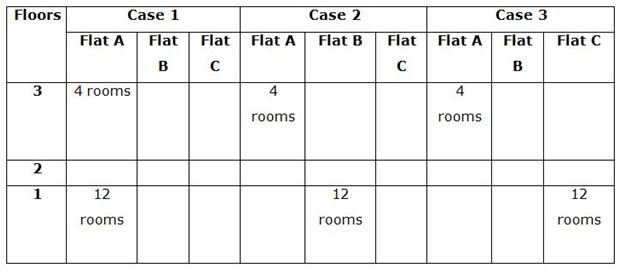• There is only one floor between the floor of 2 rooms and the floor of each room in the same flat having an area of ​​630 feet.
• So, case 1 gets eliminated.
• The flat having an area of ​​504 feet in each room is an even numbered floor.
• The flat having each room area of ​​315 ft is placed immediately below the 5 room flat.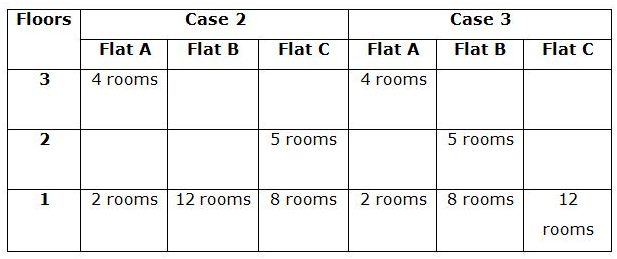• The total number of rooms in all the flats on the second floor is 15.
• Number of rooms in second floor flat C is more than the number of rooms in second floor flat A but less than the number of rooms in second floor flat B. So, case 3 ends.
• The flat with each room area of ​​420 feet is to the west of the flat, which has an area of ​​252 feet each.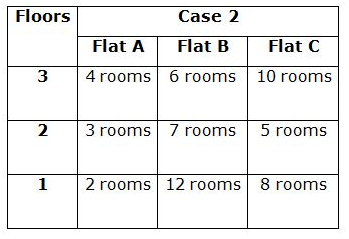Direction (4-7) :we have:

• B, who is an assistant manager, works on an even numbered floor, takes lift II.
• H who works three floors above B and is neither assistant manager nor takes the same lift as B.

That means, in case (1) H works in ICICI, and takes lift I, in case (2) H works in ICICI, and takes lift III.

• The one who is clerk from SBI takes lift I and works three floors below L, who neither works in same bank with C nor takes lift III.
• H and L have same designation but not Assistant Manager.

This means that in case (1) and case (2a) L takes lift II, in case (2b) L takes lift I.

Based on the above information we have: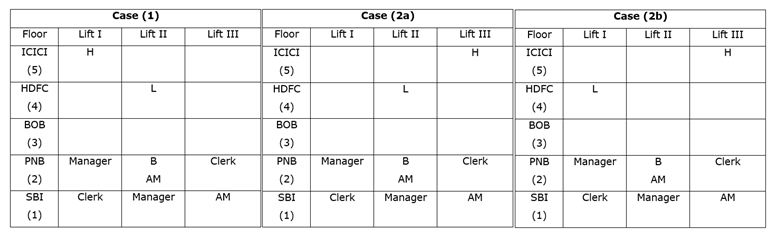Again, we have:

• E works on one of the floors below H and is designated as Manager.
• I and O work in the same bank with E, who does not take lift II.

It means that in case (1), case (2a) and case (2b) E works in BOB and takes lift III.

• L and D, who is a clerk, work in the same bank.
• The one who is manager from ICICI is not taking lift III.

Since, L is not an assistant manager and L also cannot be a clerk, both L and H must be a manager.

It means, if (1) H is designated as manager, then case (2a) and case (2b) is not valid.

Based on the above information we have: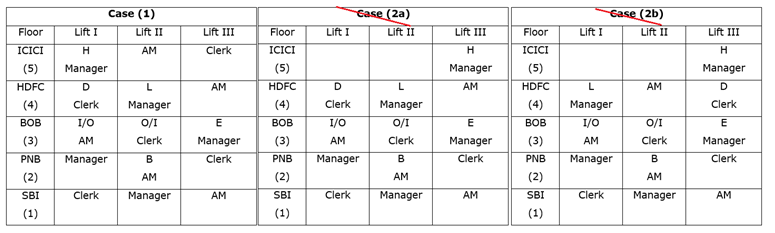Case (2A) and Case (2B) are not valid as ICICI manager is not taking lift III,

Again, we have:

• I and C have the same designation, but none of them carry lift III.
• C and K, who is an assistant manager, are working on the same floor.

It means, C is a clerk and works in SBI.

• N works on the same floor as F, who takes lift II.

It means, F takes lift II and works in ICICI.

• G works as a manager and works three floors below J, who does not take the same lift as A.

That is, G works in SBI and A works in PNB.

Based on the above information, the final arrangement we have is as follows: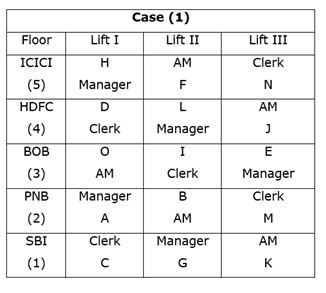Direction (8-10):

we have:

• The total number of rooms on floor number 3 is 57.
• The area of ​​each room of flat I from floor no. 3 is 94.5 m. Is2,

i.e. number of rooms from flat I to floor no. 3 = 2268/94.5 = 24.

• The number of rooms in flat II of floor 2 is 3/4. Isth From the number of rooms in flat I of floor no. 3

Thus, number of rooms in flat II of floor no. 2 = 24 x 3/4 = 18.

Based on the above information we have: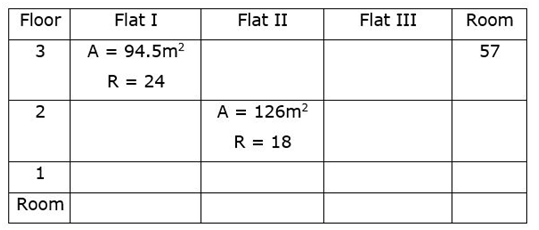Again, we have:

• The area of ​​each room from flat II of floor 3 is 63 sq.m.2 The area of ​​each room is greater than that of flat II on floor no.

Thus, area of ​​each room from flat II of floor 3 = 189m2,

It means, number of rooms in flat II of floor 3 = 2268/189 = 12

Since, total number of rooms from floor 3 = 57

Thus, number of rooms in flat III of floor 3 = 57 – 36 = 21.

Area of ​​each room from floor 3 of flat III = 2268/21 = 108m2,

• The area of ​​each room from flat III of floor number 3 is twice the number of rooms in flat III of floor no.

Thus, number of rooms in flat III of floor no.1 = 54.

• The number of rooms from flat I of floor 2 is equal to the area of ​​each room from flat III of floor 1.

Thus, the number of rooms in flat I on floor 2 = 42.

• The number of rooms in the flat in which I have taken all the floors together is 72.

Thus, number of rooms in flat I of floor 1 = 72 – (42 + 24) = 6

• The number of rooms in flat I of floor no.1 is the square root of the number of rooms in flat-III of floor no.2.

Thus, number of rooms in flat III of floor 2 = 36.

Based on the above information we have: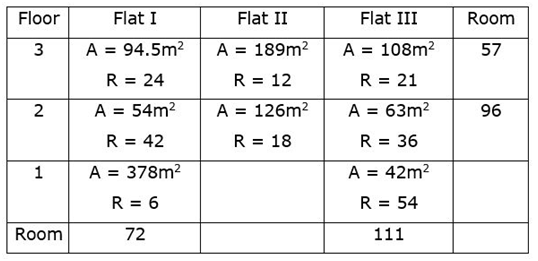Again, we have:

• The total number of rooms in Flat I is 14 more than the total number of rooms in Flat II.

Thus, number of rooms in flat II = 72 – 14 = 58

i.e., number of rooms in flat II of floor 1 = 58 – (12 + 18) = 28

Based on the above information, the final arrangement we have is as follows: